# js实现贝塞尔曲线，div也能如此丝滑？

2023 年 01 月 05 日

css
Js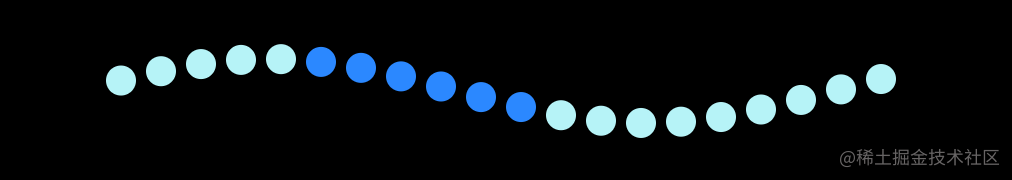### 实现

#### 布局

``````<template>
<div class="container">
<div class="content">
<span class="circle" v-for="(item,index) in list" :key="index"></span>
</div>
</div>
</template>
``````

#### Math.sin() 和 Math.cos()

Math.sin(x)      x 的正玄值。返回值在 -1.0 到 1.0 之间；

Math.cos(x)    x 的余弦值。返回的是 -1.0 到 1.0 之间；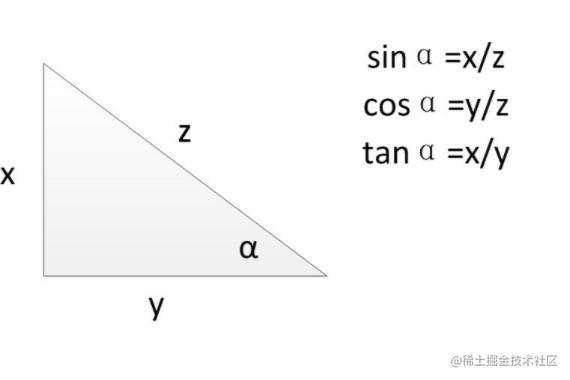sin(∠A) = 对边比斜边（a / c）
cos(∠A) = 临边比斜边 （b / c）

#### 正弦曲线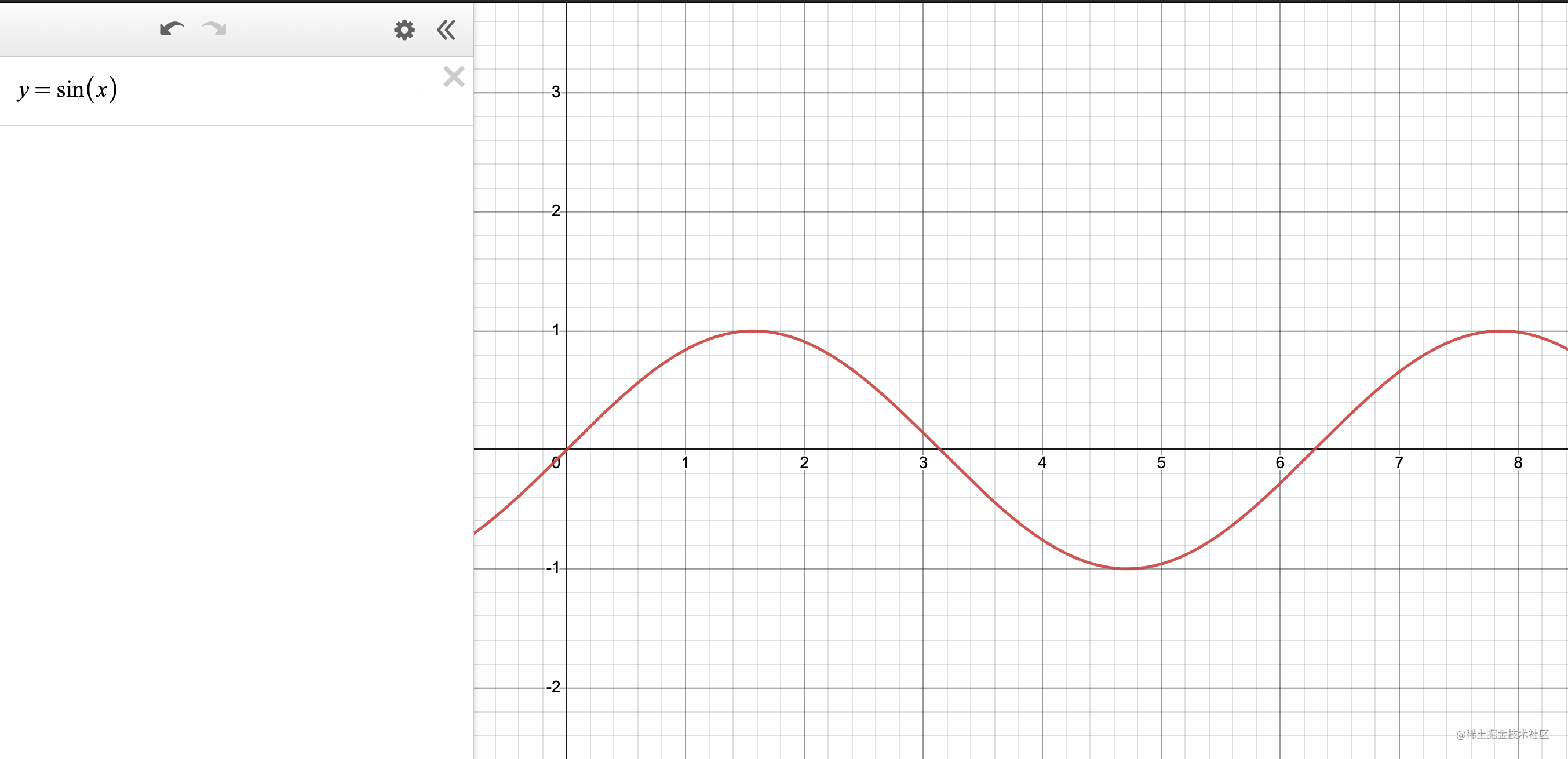#### 余弦曲线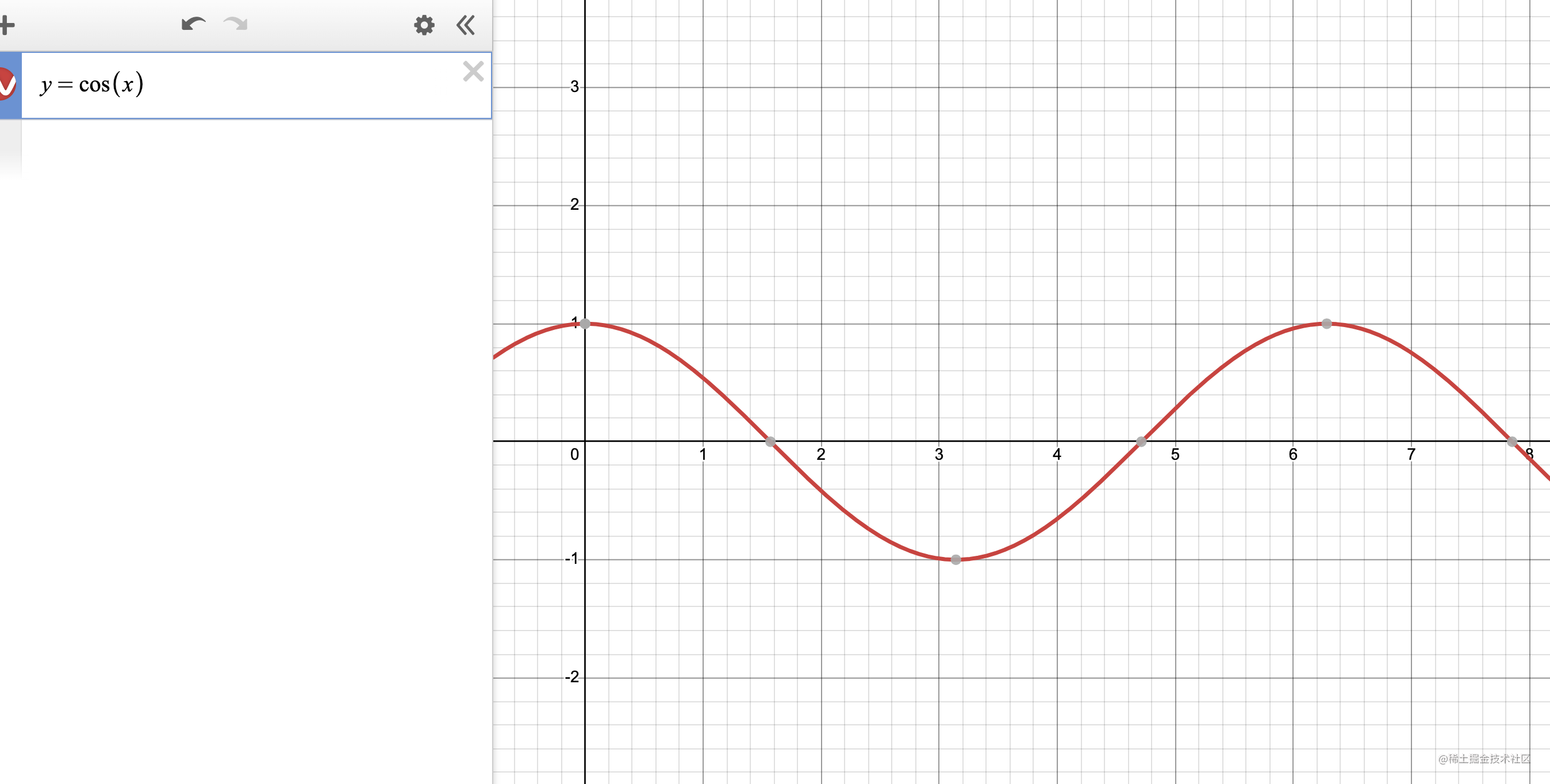1. 在曲线中 y = cos(x)

2. 在曲线中，曲线的宽度是

3. 在曲线中，曲线的高度最高点到最低点是2

4. 在我们的需求中，总宽度是400px

5. 在我们需求中， 共有二十个圆圈，所以我们可以算出每个球的宽度平均是20px，所以坐标就是`(index+1)*20`

现在我们知道了很多信息，我们就可以计算出更多信息了

#### 计算更多信息

1. 我们知道曲线的宽度和我们的物理实际宽度就可以得出宽度比： 400 / 2π

2. 这个时候我们需要通过这个比例计算出物理的x坐标对应的曲线中的x坐标，那么 物理宽度/x坐标 = 2π/曲线中x坐标

``````/* 400 / x = 2π / y, 我们的x是已知的，等下自己可以拿，这样拿到了曲线中实际的x坐标 */
const z = 400 x / 400 * Math.PI*2
``````
3. 有个曲线中的对应x坐标，通过公式我们就可以拿到其曲线中实际y坐标了

``````/* 这样就拿到了曲线中的y坐标 */
y = Math.sin(z)
``````
4. 拿到了曲线中的y坐标，那么们又知道，曲线中的总高是2，通过xy的坐标对比，我们可以计算出我们所需的真实的y

``````/* 真实宽度400/曲线宽度2π = 真实高度y/曲线中的y 通过对比得到真实的y点 */
Y = Math.sin(z) * 400 / Math.PI * 2 / 2
``````

然后通过这样的一个计算公式把这个y值赋值给我们的y点就可以得到这样的曲线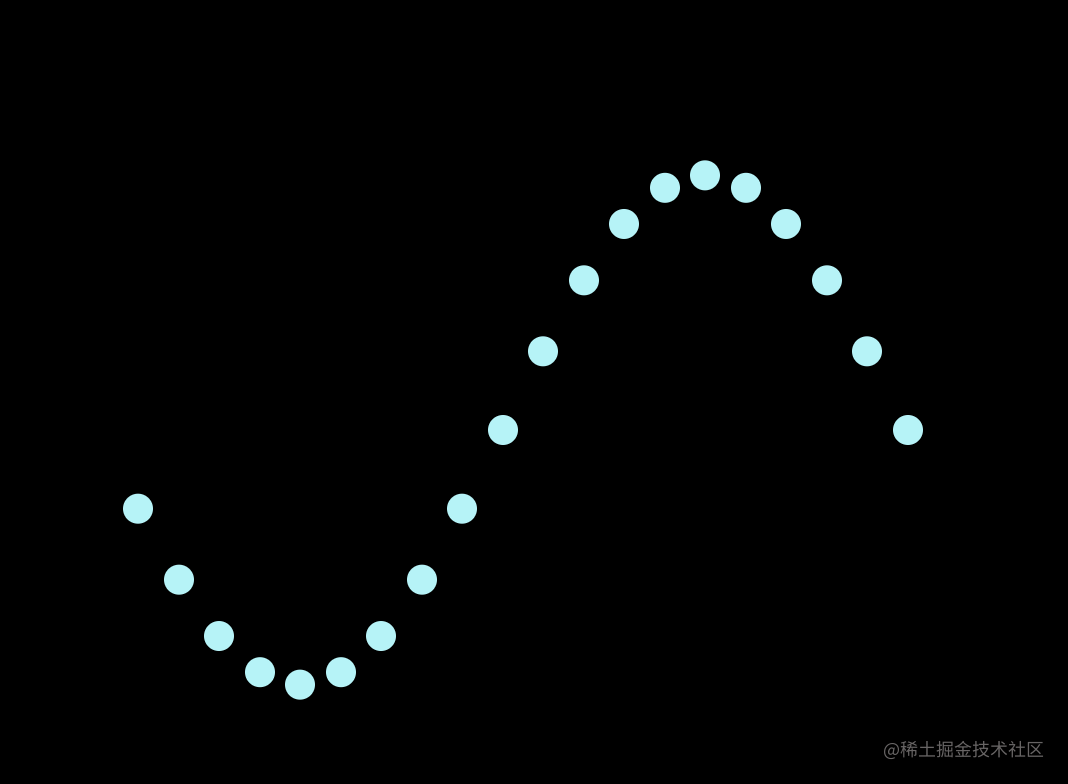### 完善剩余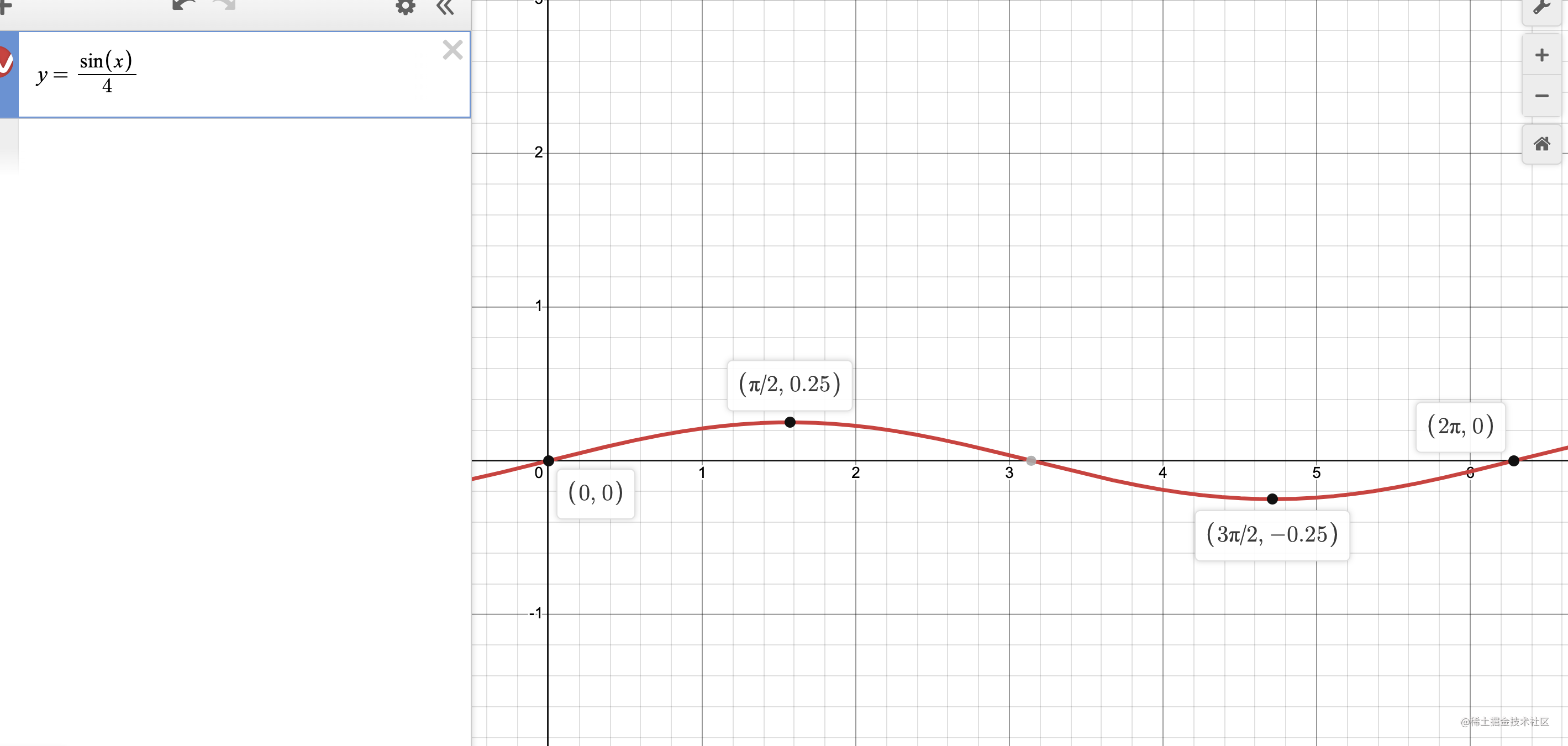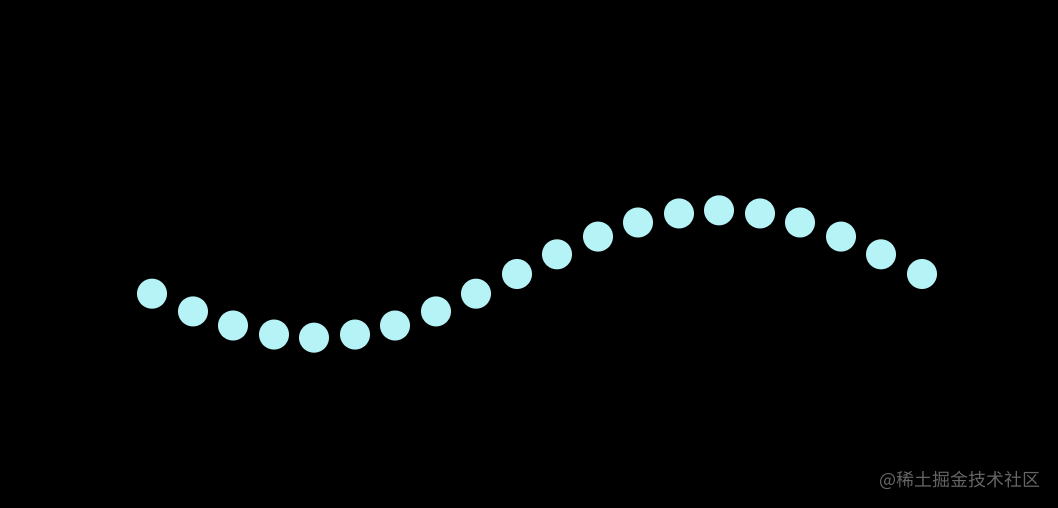### 完成跑马灯制作

``````createActiveIndex(len = 6){
return Array.from({length:len}, (v,k) => k)
},
``````

``````changeIndex(){
this.activeIndex = this.activeIndex.map( item => item === this.list.length - 1 ? 0 : item + 1)
},
``````### 贝塞尔曲线

#### 简单封装一下方法

`js中π就是Math.PI`

``````function getCoordinate(width, count,  mag = 1){
/* 通过总宽和个数计算出一个单个的宽 */
const singleWidth = width / count
/* 通过物理宽度/曲线周长计算出比率 */
const ratio = 400 / Math.PI*2
/* 上面实例代码我们是动态一次计算一个，而现在是方法，我们应该一次去拿到所有，所以我们返回一个数组对象记录xy */
let result = new Array(count).fill({})
/* 遍历总长度的dom个数，在数组中填充宽高 */
result = result.map( (item,index) => {
/* x的坐标 */
const x = (index + 1) * singleWidth
/* 定义变量z计算曲线中x的坐标 */
const z = x / width * Math.PI*2
/* 计算出真实的y的坐标 */
let y = Math.sin(z) / 4  * 400 / Math.PI * 2 / 2
/* y还需要通过倍率改变曲线，得到最终我们想要的y */
y = y / mag
/* 写入数组对象中 */
return {x, y}
})
return result;
}
``````

2023 年 01 月 05 日

0

Like

0